# 751.33 Concrete Semi Deep Abutments

## 751.33.1 General

### 751.33.1.1 Material Properties

Concrete
Class B Concrete (Substructure)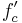$\, f'_c$ = 3.0 ksi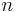$\, n$ = 10 ksi
Class B-2 Concrete (Abutment Slab)$\, f'_c$ = 4.0 ksi$\, n$ = 8 ksi

Modulus of elasticity,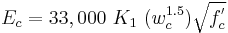$E_c = 33,000\ K_1 \ (w_c^{1.5}) \sqrt{f^'_c}$

Where:

f'c in ksi
wc = unit weight of nonreinforced concrete = 0.145 kcf
K1 = correction factor for source of aggregate
= 1.0 unless determined by physical testing

Modulus of rupture,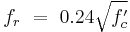$f_r \ = \ 0.24 \sqrt{f'_{c}}$       LRFD 5.4.2.6

Where:

f'c is in ksi

Reinforcing Steel
Minimum yield strength,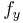$\, f_y$ = 60.0 ksi
Steel modulus of elasticity,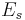$\, E_s$ = 29000 ksi

## 751.33.2 Design

### 751.33.2.1 Limit States and Factors

In general, each component shall satisfy the following equation: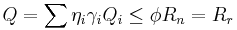$\, Q = \sum \eta_i \gamma_i Q_i \le \phi R_n = R_r$

Where: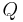$\, Q$ = Total factored force effect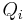$\, Q_i$ = Force effect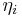$\, \eta_i$ = Load modifier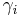$\, \gamma_i$ = Load factor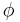$\, \phi$ = Resistance factor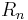$\, R_n$ = Nominal resistance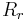$\, R_r$ = Factored resistance

Limit States

The following limit states shall be considered for abutment design:

STRENGTH – I
STRENGTH – III
STRENGTH – IV
STRENGTH – V
SERVICE – I
FATIGUE
EXTREME EVENT - II

See LRFD Table 3.4.1-1 and LRFD 3.4.2 for Loads and Load Factors applied at each given limit state.

Resistance factors

STRENGTH limit states, see LRFD 6.5.4.2 and LRFD 5.5.4.2
For all other limit states,$\, \phi$ = 1.00

Loads from stringers/girders shall be applied as concentrated loads at the intersection of the centerline of stringer/girder and the centerline of the bearing. Loads from Abutment components (Abutment Slab, Barrier Curb, Wings, Apron, Curtain Wall, etc) shall be applied to the Bearing Beam and Transverse Approach Beam respectively as uniform distributed loads for the computation of pile loads and reinforcement.

Loads from stringers/girders shall be applied as concentrated loads at the intersection of the centerline of stringer/girder and the centerline of the bearing. Loads from the Abutment Slab shall be applied to the Transverse Approach Beam as a uniform distributed load for the computation of pile loads and reinforcement. No Live load shall be distributed through the Abutment Slab to the Bearing Beam for computations of pile loads or reinforcement

These loads shall be disregarded, except for special cases or if specified by the Structural Project Manager, or on the Design Layout Sheet.

Earth pressure loads are applied to the apron wall and part of the wing wall that extends past the transverse approach beam respectively. All tables have these forces already incorporated. For all special designs, these forces shall be applied appropriately.(*) Or compute using the$\, \phi$ angle, when it is given

Note: Do not use earth pressure on the front faces.

Elevation

### 751.33.2.3 Design Assumptions

Abutment Slab

Assume a uniform 3” wearing surface load. Assume slab to be acting one-way parallel with roadway.

Bearing Beam

The beam is assumed continuous over supports at centerline of piles. Live loads are applied to the superstructure only. Live loads are not simultaneously applied to the abutment slab.

Transverse Approach Beam

The beam is assumed continuous over supports at centerline of piles. The beam is assumed to carry half of the dead load of the approach slab. Live loads are applied to the abutment slab only. Live loads are not simultaneously applied to the approach slab.

Apron Wall

Assume apron wall to be cantilevered off of bearing beam with no support from battered piles. Use an equivalent fluid pressure of 45 pcf.

Wing Wall

Assume wing wall to be cantilevered at the fill face side of the transverse approach beam.

Assume 1’-0” of soil braces wing wall bottom at short end of wall.

Use an equivalent fluid pressure of 45 pcf.

Include a collision impact load applied to the barrier curb transferred to the wing.

Assume LL surcharge lateral load of 90 pcf.

Piles

Stresses in the piles due to bending need not be considered in design calculations, except for seismic design.

### 751.33.2.4 Design Process

There are many ways to go about designing a Semi-Deep Abutment. Below is one process, which will work for most standard abutments. A majority of the steps are interchangeable, and can be switched to meet the designers’ preference.

Design geometry of abutment
Calculate the size and number of piles required for the bearing beam
Determine the bearing beam reinforcement
Calculate the size and number of piles required for the transverse beam
Determine the other abutment components reinforcement

## 751.33.3 Geometry

Use the standard illustrations in this section, information from your superstructure calculations, and the design layout sheet to design the geometry of the abutment.

### 751.33.3.1 Wing Elevation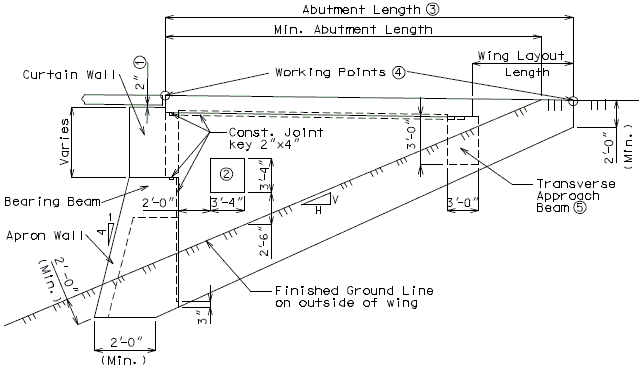Wing ElevationTop of curtain wall to be 2” below the slab of the adjoining span.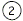An access door shall be used only when called for on the Design Layout Sheet (based on utility need or removal of form need).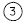It may be necessary to increase Abutment Length so end of wing extends beyond the intersection of the ground line and top of slab at the outside edge of wing.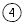Working points shall include elevations at grade for the bridge.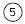Slope Transverse Beam both top and bottom when the Abutment Slab haunch exceeds 6” (at gutter line).

### 751.33.3.2 Cross-SectionCross-SectionCheck the profile sheet with Design Layout for the slope of finish ground line.Check the clearance of anchor bolt wells; increase beam depth if needed.If length is greater than 15’-0”, Apron wall reinforcement shall be designed.Abutment Slab Depth + 1/2” (Min.)12” (Min.) at gutter line to the top of the Transverse Approach Beam, except when the Abutment Slab depth is 12” or greater, then place the top of the Transverse Approach Beam 1/2” below the bottom of the slab. (*) Provide a minimum of 8" cl. from outside edge of pile to face of beam.

### 751.33.3.3 Plan View

Square Structures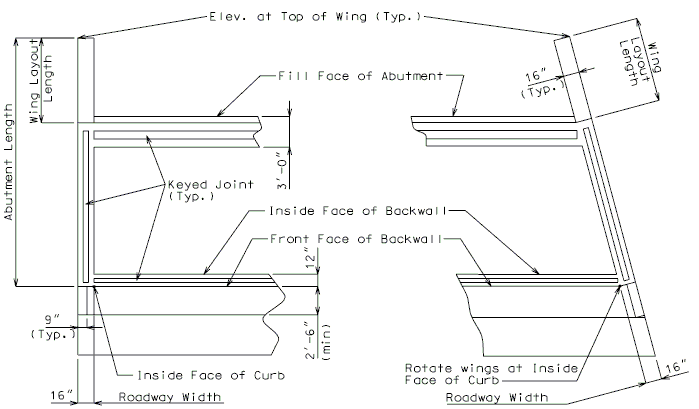### 751.33.3.4 Front Elevation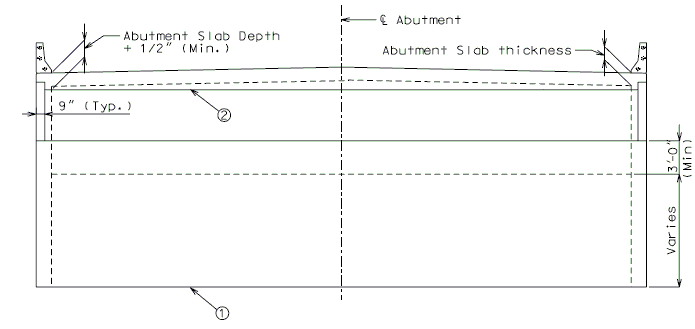Front ElevationSlope toe of Apron Wall when the ends of the walls differ in elevations by more than 2’-0”. (or when beam is sloped)Slope top of backwall when the slab haunch exceeds 6” (at gutter line).

### 751.33.3.5 Pile Location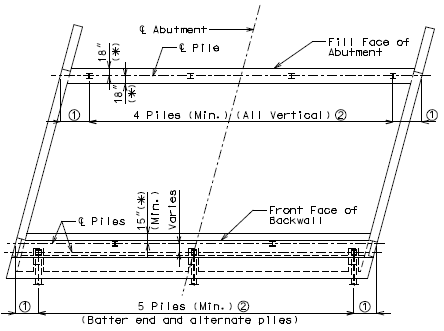Pile Location21” (Min.), 2’-0” (Max).30” or 2.5 x pile diameter (Min.), 12’-0” (Max.) spacing, 1” increments. (*) Provide a minimum of 8" cl. from outside edge of pile to face of beam.

## 751.33.4 Reinforcement

### 751.33.4.1 Front Elevation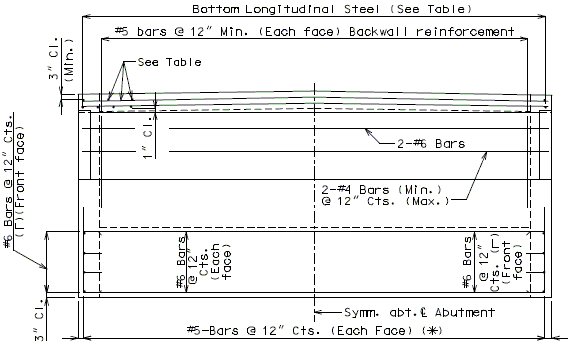Front Elevation

See Table below for reinforcement not shown.

All transverse steel to be placed parallel to the backwall. All main steel to be placed parallel to the centerline of roadway.

Epoxy coat all reinforcing in abutment slab, bearing beam, curtain wall, apron wall, backwall, and transverse approach beam.

When expansion devise is present use details of protective coating and sloping top of beam to drain.

(*) If height of Equivalent Fluid Pressure A is greater than 5 ft. or if apron wall extends more than 15 ft. below bearing beam, reinforcement will need to be verified.

### 751.33.4.2 Wing Elevation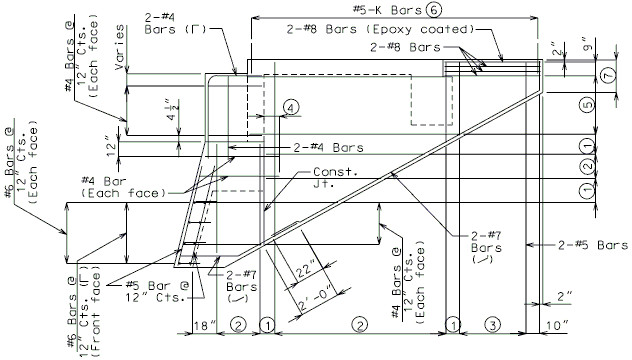Wing ElevationVaries (12” Max.)#4 Bars at 12” cts. (Each face)#5 Bars at 12” cts. (Each face)12” (Min.) (Development length)#6 Bars at 8” cts. (Each face)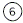See barrier curb details and spacing of K bars.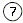If end of wing wall is greater than 5’-0” deep, reinforcement will need to be verified.

### 751.33.4.3 Cross-Section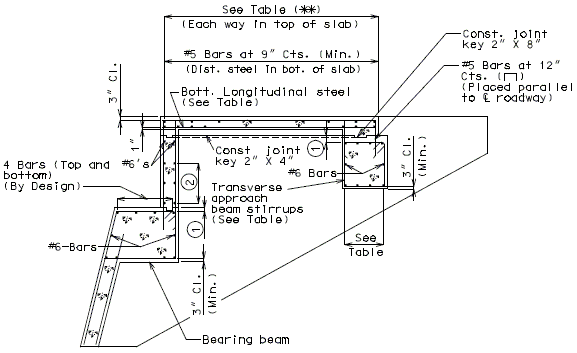Cross Section

 See Table below for reinforcement.2 1/2" Cl. (To clear the const. joint key).#4 @ 12" cts. (Max).

 AbutmentSlab DesignLengths (*) UniformAbutmentSlabThickness AxleVehicleLive Load(***) AbutmentSlab BottomSteelParallel toC.L. Rdwy. AbutmentSlab TopSteel EachWay Transverse Approach Beam Reinforcement(Top & Bot.) (****) Pile Spacing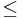$\, \le$ 7' 8' 9' 10' 11' 12' 10'-0" 11" 40.00 k #8 at 9" cts. #4 at 18" cts. 4-#6 4-#6 4-#6 4-#6 4-#7 4-#8 15'-0" 12" 43.33 k #8 at 8" cts. #4 at 12" cts. 4-#6 4-#6 4-#6 4-#7 4-#7 4-#8 20'-0" 15" 45.00 k #8 at 7" cts. #4 at 12" cts. 4-#6 4-#6 4-#7 4-#7 4-#8 4-#8 25'-0" 17" 46.08 k #8 at 5" cts. #4 at 12" cts. 4-#6 4-#6 4-#7 4-#7 4-#8 6-#8 30'-0" 20" 49.60 k #9 at 6" cts. #5 at 12" cts. 4-#6 4-#7 4-#7 4-#8 6-#8 6-#8 35'-0" 22" 52.80 k #9 at 5" cts. #5 at 12" cts. 4-#6 4-#7 4-#8 4-#8 6-#8 6-#8 Transverse Approach Beam Stirrup Size #4 #4 #5 #5 #6 #6

(*) Abutment slab design length is measured from the center of the backwall to the center of the approach beam along the centerline of roadway.

(**) For abutment slabs with lengths greater than 35’-0”, an intermediate bent is required inside the semi-deep abutment. Top and bottom reinforcement for these slabs shall be designed by the designer.

(***) Use the axle vehicle live load for calculating pile loads for the transverse approach beam.

(****) 4-#9’s can be used in lieu of 6-#8’s if spacing restrictions require fewer bars.

### 751.33.4.4 Plan View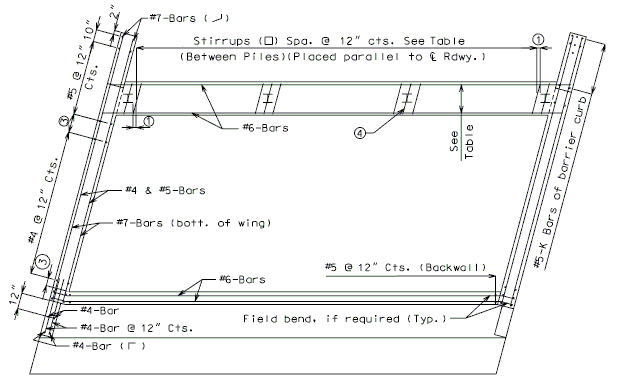Section at Upper Construction Joint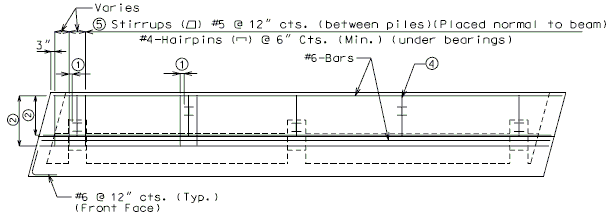Plan of Bearing Beam below Lower Keyed Construction Joint

 See table above for reinforcement not shown.Use 3” (min.) and 6” (max.) (Typ.).See EPG 751.33.4.5Varies (12” max.)Stirrup to be centered over pile (Typ.).If nominal pile capacity is greater than 110 tons, stirrup size and spacing needs to be verified.

### 751.33.4.5 Bearing Beam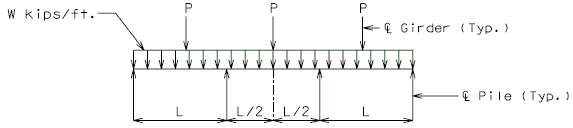Basic Assumption (Continuous Beam)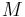$\, M$ =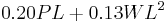$\, 0.20PL + 0.13WL^2$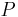$\, P$ = Superstructure Loads (Applied through beam seat)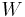$\, W$ = Uniform DL (Abutment Components)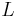$\, L$ = Pile Spacing (ft.)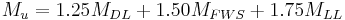$\, M_u = 1.25 M_{DL} + 1.50M_{FWS} + 1.75M_{LL}$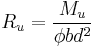$\, R_u = \frac {M_u}{\phi bd^2}$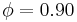$\, \phi = 0.90$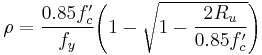$\, \rho = \frac{0.85 f'_c}{f_y} \Bigg( 1 - \sqrt {1 - \frac {2R_u}{0.85 f'_c}} \Bigg)$

 Min. Reinforcement: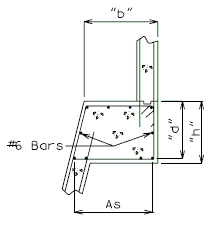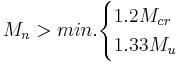$\, M_n > min. \begin{cases} 1.2M_{cr} \\ 1.33M_u \end{cases}$ Crack Control Parameters: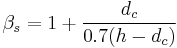$\, \beta_s = 1 + \frac {d_c}{0.7(h-d_c)}$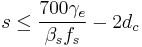$\, s \le \frac {700 \gamma_e}{\beta_s f_s} - 2d_c$

See LRFD 5.7.3.4 for variable definitions.

### 751.33.4.6 Pile HaunchesPart Section Thru Bearing Beam
(*) Provide appropriate notes for steel H pile and steel pile shells.Plan of Bearing Beam below lower construction joint
(Showing typical dimensions for different pile types)

## 751.33.5 Details

### 751.33.5.1 Front Sheet

Figure shown below is an example of the information to be included on the abutment plan view on the first sheet of the plans.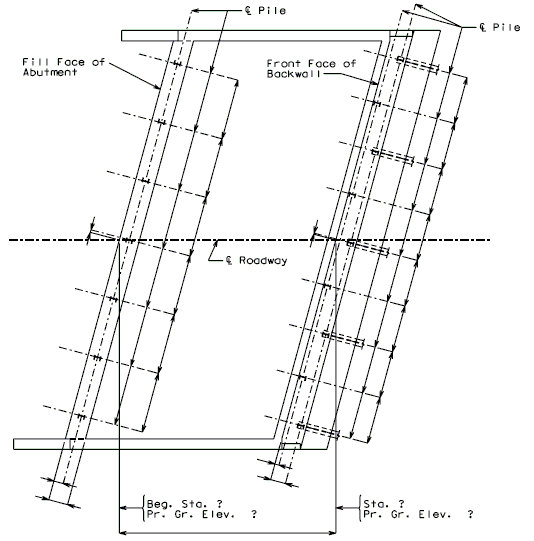Plan View

Details for unsymmetrical roadways will require dimensions tying centerline of roadway to centerline of structure. (Pile coordinates shall be to centerline of roadway)

### 751.33.5.2 Ground line within Abutments

Figure shown below shall be detailed on the first or second sheet of the plans.Ground Line and Piling in Abutments

### 751.33.5.3 Construction Joints(*) When the total length of beam and backwall exceeds 60'-0", use keyed construction joint at or near centerline Abutment as shown, perferably located at a 1/4 point between pilesConstruction Joints

### 751.33.5.4 Access Door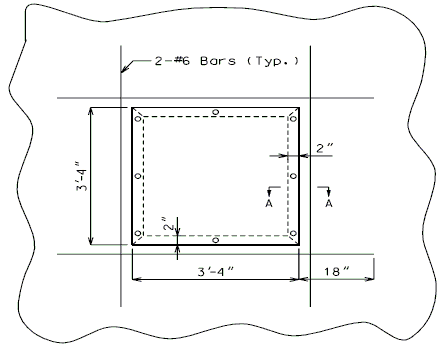Door Elevation

Cost of the door is to be included in other items.
Cut or bend reinforcing steel in the field to clear the opening for the access door.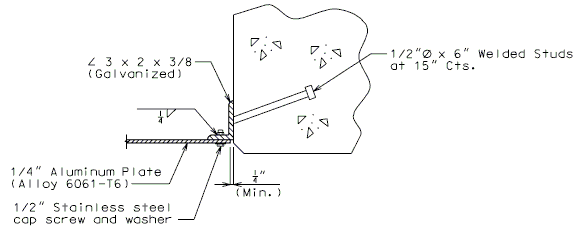Section A-A